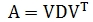# SQL Server eigenvalues and eigenvectors

SYMMEIG

Updated: 10 May 2016

Use the table-valued function SYMMEIG to return the D and V matrices representing the eigenvalues and eigenvectors of a real symmetric matrix. The input into the function is an SQL statement having a resultant table which is a real, symmetric matrix. The resultant table can be in either 3rd-normal or 'spreadsheet' form. SYMMEIG returns a table in third normal form containing the D and V matrices such that:Syntax
SELECT * FROM [wct].[SYMMEIG](
<@MatrixQuery, nvarchar(max),>
,<@Is3N, bit,>)
Arguments
@MatrixQuery
An SQL statement which upon execution returns a real symmetric matrix or the string representation of the matrix with the columns separated by commas and the rows separated by semicolons.
@Is3N
A bit value which indicates whether the resultant table returned by @MatrixQuery is in 3rd-normal form. Enter TRUE for a resultant table in 3rd-normal form.
Return Types
TABLE (
[RowNum] [int] NULL,
[ColNum] [int] NULL,
[Value] [float] NULL,
[Type] [nvarchar](2) NULL
)
Remarks
·         If @Is3N is NULL then @Is3N = FALSE
·         [Type] is either 'D' or 'V'
·         Available in XLeratorDB / math 2008 only
Examples
Example #1
In this example we supply @A as a real symmetric matrix and return the D and V matrices.
DECLARE @A as varchar(max) = '5,15,55,225;15,55,225,979;55,225,979,4425;225,979,4425,20515'
SELECT * FROM wct.SYMMEIG(@A,NULL)

This produces the following result.

Example #2
Using the same values, with the matrix passed in as SQL which produces a resultant table not in 3rd-normal form.
SELECT * FROM wct.SYMMEIG('SELECT * FROM (VALUES (5,15,55,225),(15,55,225,979),(55,225,979,4425),(225,979,4425,20515))n(x1,x2,x3,x4)','False')
This produces the following result.

Example #3
Using the same values, with the matrix passed in as SQL which produces a result in 3rd-normal form.
SELECT *
INTO #t
FROM (VALUES
(0,0,5)
,(0,1,15)
,(0,2,55)
,(0,3,225)
,(1,0,15)
,(1,1,55)
,(1,2,225)
,(1,3,979)
,(2,0,55)
,(2,1,225)
,(2,2,979)
,(2,3,4425)
,(3,0,225)
,(3,1,979)
,(3,2,4425)
,(3,3,20515)
)n(r,c,x)

SELECT * FROM wct.SYMMEIG('SELECT * FROM #t','True')

This produces the following result.

### SupportCopyright 2008-2023 Westclintech LLC         Privacy Policy        Terms of Service